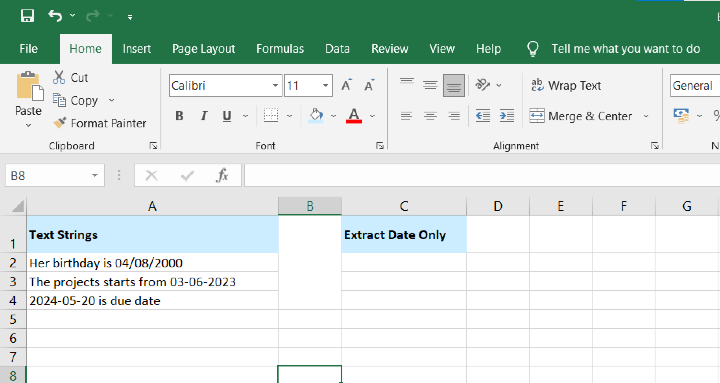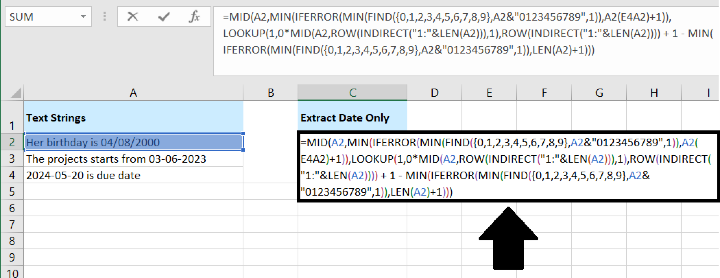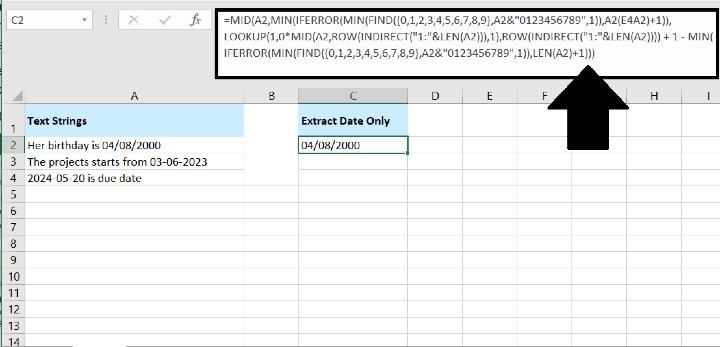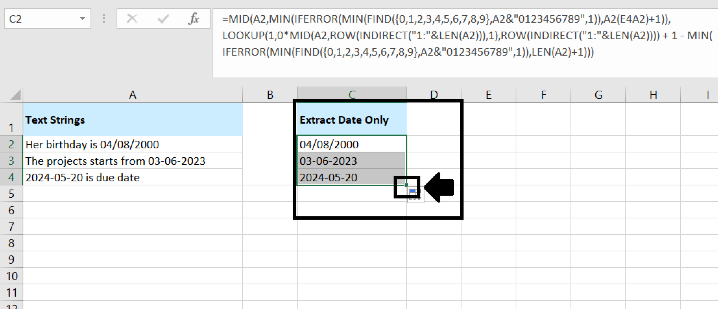# How To Extract Date From Text Strings In Excel?

Excel is a powerful tool that allows users to manipulate and analyze data efficiently. However, working with text strings containing dates can sometimes be challenging. Extracting dates from text strings is a common requirement when dealing with datasets that include unstructured data. In this article, we will explore various techniques and formulas to extract dates from text strings in Excel, enabling you to work with dates more effectively.

If you need to extract only the date from a list of text strings in Excel, you can use the following array formula. Follow these steps to achieve the desired result−

To extract only the date from a text string in Excel, follow these steps−• Select an empty cell where you want the result to appear.

• Enter the formula provided below into the selected cell−

=MID(A2,MIN(IFERROR(MIN(FIND({0,1,2,3,4,5,6,7,8,9},A2&"0123456789",1)),LEN(A2)+1)),LOOKUP(1,0*MID(A2,ROW(INDIRECT("1:"&LEN(A2))),1),ROW(INDIRECT("1:"&LEN(A2))))+1−MIN(IFERROR(MIN(FIND({0,1,2,3,4,5,6,7,8,9},A2&"0123456789",1)),LEN(A2)+1)))
• Press Enter.Once you press Enter, the formula will extract the date from the text string and display it in the selected cell. Refer to the accompanying screenshot for a visual representation of the extracted date.

After entering the formula into a cell and pressing Enter to extract the date from a text string in Excel, you can easily apply the formula to other cells using the fill handle. Here's how −

• Select the cell with the formula.

• Click and hold the fill handle (a small square at the bottom−right corner of the selected cell).

• Drag the fill handle down to the cells where you want to apply the formula.

• Release the mouse button.

By dragging the fill handle, the formula will automatically adjust to the corresponding cells, extracting dates from their respective text strings. Refer to the provided screenshot for a visual representation of the extracted results.By following these steps, you can easily extract the date from a text string in Excel, enabling you to work with the extracted dates for further analysis or calculations.

## Conclusion

Extracting dates from text strings can be essential for performing calculations and analysis in Excel. By using the techniques mentioned above, you can effectively extract dates from text strings, regardless of the format in which they appear. Whether you choose to utilize Excel's built−in text functions or leverage the power of regular expressions, these methods will enable you to work with dates more efficiently and accurately in your Excel spreadsheets.

Updated on: 13-Jul-2023

663 Views